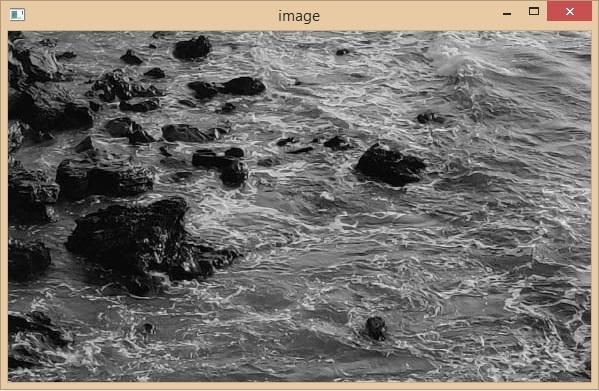# OpenCV Python program to read and save an Image

OpenCV-python is an Open Source Computer Vision Library in python it is used to process images and videos for detecting faces and objects. It is one of the image processing libraries in python and uses the python Numpy library so that all the image arrays are represented as a ndarray type.

### Install OpenCV using pip

Pip install opencv-python


OpenCV needs the numpy library, we need to make sure that the numpy library is also installed. The Python OpenCV module provides functions cv2.imread() and cv2.imwrite() to read/load and write/save image files.

### Syntax

Cv2.imread(filename[, flags])


### Parameters

• Filename: Name/destination of a file that has to be loaded.

• Flags: Flag that can take values of ImreadModes, that specifies the color type of a loaded image

### Return Value

• The method returns a Numpy array. If the image cannot be read (because of a missing file, improper permissions, or unsupported/invalid format), this function will return an empty matrix instead of generating the error.

The function decides the type of an image by using the content, not by the extension.

### Supported file formats

• Windows bitmaps: *.bmp, *.dib

• JPEG files: *.jpeg, *.jpg, *.jpe

• JPEG 2000 files: *.jp2

• Portable Network Graphics: *.png

• WebP: *.webp

• Portable image format: *.pbm, *.pgm, *.ppm *.pxm, *.pnm

• Sun rasters: *.sr, *.ras

• OpenEXR Image files: *.exr

Note: Raster and Vector geospatial data supported by GDAL

### Algorithm for read an image

• Import the cv2 module.

• Display the image in a window using the cv2.imshow() method,

• And set a timer for the output window using the cv2.waitKey(0) method.

• Finally, close the output window by using the cv2.destroyAllWindows() method.

### Example

Let’s take an image as an input.

#importing the opencv module
import cv2

# display image
cv2.imshow('image',img)

cv2.waitKey(0)
cv2.destroyAllWindows()


### Output### Example

The imread() function stores the color image in a 3D ndarray of row (height) x column (width) x color (3). Let’s take a color image as an input and read it with the imread() function.

#importing the opencv module
import cv2

# display image
cv2.imshow('image',img)
cv2.waitKey(0)
cv2.destroyAllWindows()

print('Type:',type(img))
print('Shape:',img.shape)
print('datatype',img.dtype)


### OutputType: <class 'numpy.ndarray'>
Shape: (225, 225, 3)
datatype uint8


The image object img has stored the 3-dimensional numpy array of (225, 225, 3) shape.

## The cv2.imwrite() function

The cv2.imwrite() function saves the image with a specified name. The filename extension and location are also chosen from the first parameter of the function.

This function returns a Boolean value, True will be returned if the image is written successfully. else it will return False. If the unsupported image format is specified, then the image will be converted to 8-bit unsigned (CV_8U) and saved that way.

Following is the syntax of this function –

Cv2.imwrite(filename, img[, params])


Where,

• filename: Name/destination where the file has to be saved.

• img: It takes a ndarray of values to save an image.

• params: list of Format-specific parameters encoded as pairs.

### Example

In this example, we will save an image using a zeros array of (100,100,3) shape.

#import the numpay and opencv modules
import numpy as np
import cv2

# create a 3-d numpy array
blank_image2 = np.zeros((100,100,3), dtype=np.uint8)

# save or create an image
cv2.imwrite("written_image.jpg", blank_image2)


### Output

True


The function returned the Boolean Value True which indicates the file has been successfully written/saved with the specified name. Also we can see the blank image with the name written_image.jpg in the above output block.

### Example

In this example, we will read the lenna.png image from the Image folder, then re-write it using the params parameter with the highest quality.

The matrix, [cv2.IMWRITE_JPEG_QUALITY, 100] indicates highest quality. Whereas 0 is for lowest quality and the default is 95.

#importing the opencv module
import cv2

cv2.imshow('Input image',img)
cv2.waitKey(0)
cv2.destroyAllWindows()

print('Type:',type(img))

status = cv2.imwrite('Images/Output_lenna_opencv_red_high.jpg', img, [cv2.IMWRITE_JPEG_QUALITY, 100])
print('Status:', status)


### InputType: <class 'numpy.ndarray'
Status: True
Details of the


The type of the img object is ndarray. And the imwrite() function saved the image Output_lenna_opencv_red_high.jpg in the Images folder successfully.

Updated on: 30-May-2023

401 Views